# Central Science: Chapter 14 Flashcards

Set Details Share
created 3 years ago by Chemistry4Life
2,332 views
updated 3 years ago by Chemistry4Life
College: First year, College: Second year, College: Third year, College: Fourth year
Subjects:
chemistry
Page to share:
Embed this setcancel
COPY
code changes based on your size selection
Size:
X

1

A burning splint will burn more vigorously in pure oxygen than in air because ________.
A) oxygen is a reactant in combustion and concentration of oxygen is higher in pure oxygen than is in air
B) oxygen is a catalyst for combustion
C) oxygen is a product of combustion
D) nitrogen is a product of combustion and the system reaches equilibrium at a lower temperature
E) nitrogen is a reactant in combustion and its low concentration in pure oxygen catalyzes the combustion

A

2

Of the following, all are valid units for a reaction rate except ________.
A) mol/L
B) M/s
C) mol/hr
D) g/s
E) mol/L-hr

A

3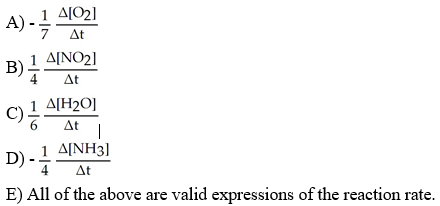Which one of the following is not a valid expression for the rate of the reaction below?

4NH3 + 7O2 → 4NO2 + 6H2O

E

4

The rate law of a reaction is rate = k[D][X]. The units of the rate constant are ________.
A) mol L-1s-1
B) L mol-1s-1
C) mol2 L-2s-1
D) mol L-1s-2
E) L2 mol-2s-1

B

5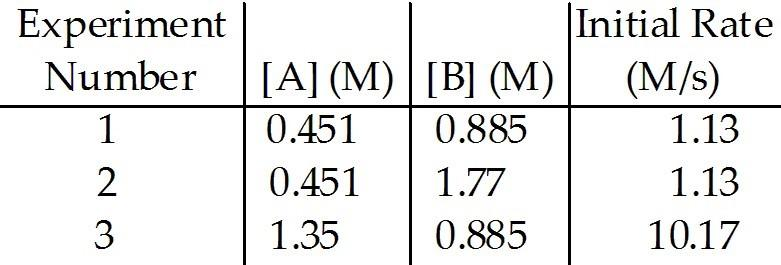The data in the table below were obtained for the reaction:

A + B → C

5) The rate law for this reaction is rate = ________.
A) k[A][B]
B) k[P]
C) k[A]2[B]
D) k[A]2[B]2
E) k[A]2

E

6The data in the table below were obtained for the reaction:

A + B → C

The magnitude of the rate constant is ________.
A) 5.56
B) 0.278
C) 13.2
D) 42.0
E) 2.21

A

7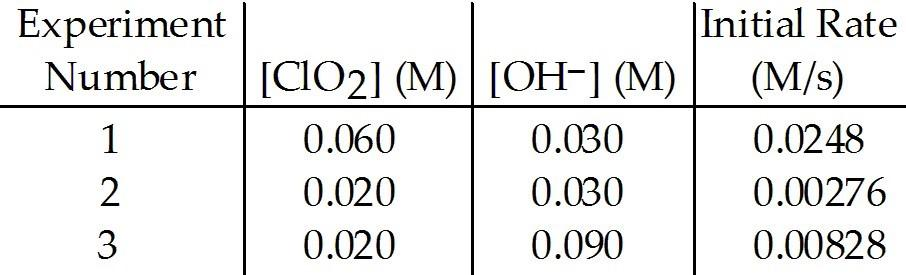The data in the table below were obtained for the reaction:

2 ClO2 (aq) + 2 OH- (aq) → ClO3- (aq) + ClO2- (aq) + H2O (1)

7) What is the order of the reaction with respect to ClO2?
A) 1
B) 0
C) 2
D) 3
E) 4

C

8The data in the table below were obtained for the reaction:

2 ClO2 (aq) + 2 OH- (aq) → ClO3- (aq) + ClO2- (aq) + H2O (1)

What is the order of the reaction with respect to OH-?
A) 0
B) 1
C) 2
D) 3
E) 4

B

9The data in the table below were obtained for the reaction:

2 ClO2 (aq) + 2 OH- (aq) → ClO3- (aq) + ClO2- (aq) + H2O (1)

Which of the following is correct regarding the overall order of the reaction?
A) the overall order is found by subtracting the individual orders for ClO2 and OH-, so it is 1 for this reaction
B) the overall order for this reaction is based on the individual order for OH-, so it is 1
C) the overall order is found by adding the individual orders for ClO2 and OH-, so it is 3 for this reaction
D) the overall order for this reaction is based on the individual order for ClO2, so it is 2
E) the overall order cannot be determined

C

10

The data in the table below were obtained for the reaction:

2 ClO2 (aq) + 2 OH- (aq) → ClO3- (aq) + ClO2- (aq) + H2O (1)

What is the magnitude of the rate constant for the reaction?
A) 1.15 × 104
B) 4.6
C) 230
D) 115
E) 713

C

11

Under constant conditions, the half-life of a first-order reaction ________.
A) is the time necessary for the reactant concentration to drop to half its original value
B) is constant
C) can be calculated from the reaction rate constant
D) does not depend on the initial reactant concentration
E) All of the above are correct.

E

12

The reaction

2NO2 → 2NO + O2

follows second-order kinetics. At 300 °C, [NO2] drops from 0.0100 M to 0.00650 M in 100.0 s. The rate constant for the reaction is ________ M-1s-1.
A) 0.096
B) 0.65
C) 0.81
D) 1.2
E) 0.54

E

13

The reaction

CH3-N≡C → CH3-C≡N

is a first-order reaction. At 230.3 °C, k = 6.29 x 10-4S-1. If [CH3-N≡C] is 1.00 × 10-3 initially, [CH3-N≡C] is ________ M after 1.000 x 103s.
A) 5.33 × 10-4
B) 2.34 × 10-4
C) 1.88 × 10-3
D) 4.27 × 10-3
E) 1.00 × 10-6

A

14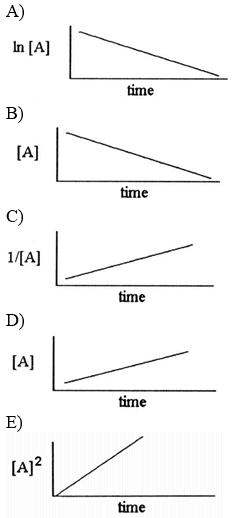Which one of the following graphs shows the correct relationship between concentration and time for a reaction that is second order in [A]?

C

15

The following reaction is second order in [A] and the rate constant is 0.025 M-1s-1:

A → B

The concentration of A was 0.65 M at 33 s. The initial concentration of A was ________ M.
A) 2.4
B) 0.27
C) 0.24
D) 1.4
E) 1.2 × 10-2

D

16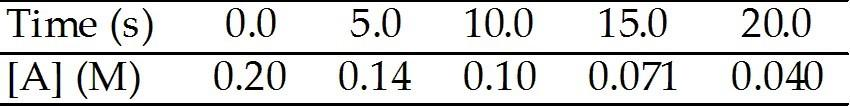The reaction A → B is first order in [A]. Consider the following data.

16) What is the rate constant (s-1) for this reaction?
A) 3.0 × 10-2
B) 14
C) 0.46
D) 4.0 × 102
E) 7.8 × 10-2

E

17The reaction A → B is first order in [A]. Consider the following data.

What is the concentration (M) of [A] after 80.0 s?
A) 4.06
B) 7.8 × 10-2
C) 5.00 × 10-3
D) 4.06 × 10-4
E) 0.01

D

18The reaction A → B is first order in [A]. Consider the following data.

The rate constant of a first-order process that has a half-life of 3.50 min is ________ s-1.
A) 0.693
B) 1.65 × 10-2
C) 1.98
D) 0.198
E) 3.30 ×10-3

E

19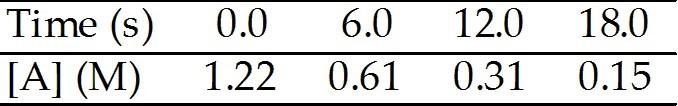The reaction A (aq) → B (aq) is first order in [A]. A solution is prepared with [A] = 1.22 M. The following data are obtained as the reaction proceeds:

The rate constant for this reaction is ________ s-1.
A) 0.23
B) 1.0
C) 0.17
D) 0.12
E) -0.12

D

20

One difference between first- and second-order reactions is that ________.
A) the half-life of a first-order reaction does not depend on [A]0; the half-life of a second-order reaction does depend on [A]0
B) the rate of both first-order and second-order reactions do not depend on reactant concentrations
C) the rate of a first-order reaction depends on reactant concentrations; the rate of a second-order reaction does not depend on reactant concentrations
D) a first-order reaction can be catalyzed; a second-order reaction cannot be catalyzed
E) None of the above are true.

A

21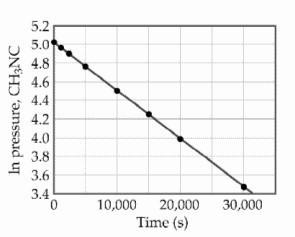At elevated temperatures, methylisonitrile (CH3NC) isomerizes to acetonitrile (CH3CN):

CH3NC (g) → CH3CN (g)

The reaction is first order in methylisonitrile. The attached graph shows data for the reaction obtained at 198.9 °C.

What is the rate constant (s-1) for the reaction?
A) -1.9 × 104
B) +5.2 × 10-5
C) +1.9 × 104
D) -5.2 × 10-5
E) +6.2

B

22

The decomposition of [A] in solution at 55 °C proceeds via first order:

A (aq) → B (aq)

What is the rate law for the reaction?
A) rate = k[A]2
B) rate = k[A]
C) rate = k [A]/[B]
D) rate = k[A][B]
E) rate = 2k[A]

B

23

As the temperature of a reaction is increased, the rate of the reaction increases because the ________.
A) reactant molecules collide less frequently
B) reactant molecules collide more frequently and with greater energy per collision
C) activation energy is lowered
D) reactant molecules collide with greater energy
E) reactant molecules collide more frequently

B

24

The rate of a reaction depends on ________.
A) collision frequency
B) collision energy
C) collision orientation
D) all of the above
E) none of the above

D

25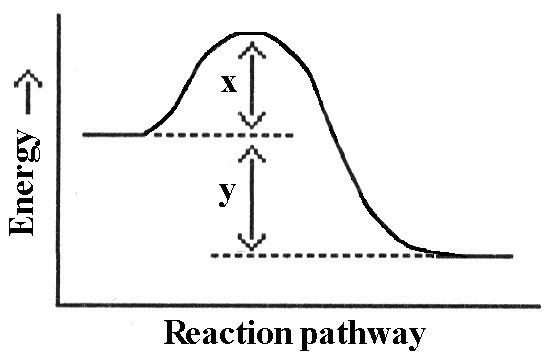Which energy difference in the energy profile below corresponds to the activation energy for the forward reaction?

A) x
B) y
C) x + y
D) x - y
E) y - x

A

26

In the energy profile of a reaction, the species that exists at the maximum on the curve is called the ________.
A) product
B) activated complex
C) activation energy
D) enthalpy of reaction
E) atomic state

B

27

In the Arrhenius equation,

k = Ae-Ea/RT

________ is the frequency factor.
A) k
B) A
C) e
D) Ea
E) R

B

28

In general, as temperature goes up, reaction rate ________.
A) goes up if the reaction is exothermic
B) goes up if the reaction is endothermic
C) goes up regardless of whether the reaction is exothermic or endothermic
D) stays the same regardless of whether the reaction is exothermic or endothermic
E) stays the same if the reaction is first order

C

29

In general, as activation energy increases, reaction rate ________.
A) goes down if the reaction is exothermic
B) goes down if the reaction is endothermic
C) stays the same regardless of whether the reaction is exothermic or endothermic
D) goes down regardless of whether the reaction is exothermic or endothermic
E) none of the above

D

30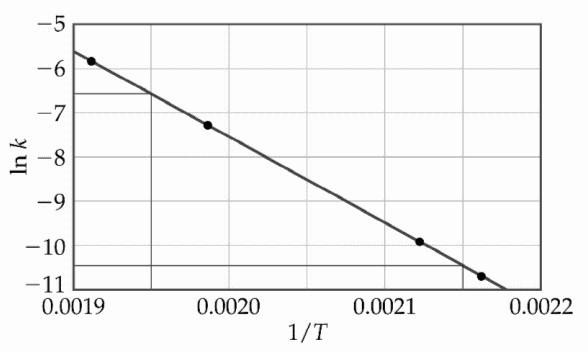The decomposition of [A] in solution at 80 °C proceeds via the following reaction:

A (aq) → B (aq)

The dependence of the rate constant on temperature is studied and the graph below is prepared from the results.

What is the energy of activation (kJ/mol) for this reaction?
A) 1.6 × 105
B) 4.4 × 10-7
C) 160
D) 4.4 × 10-4
E) 1.9 × 104

C

31

The mechanism for formation of the product X is:

A + B → C + D (slow)
B + D → X (fast)

The intermediate reactant in the reaction is ________.
A) A
B) B
C) C
D) D
E) X

D

32

For the elementary reaction

NO3 + CO → NO2 + CO2

the molecularity of the reaction is ________, and the rate law is
A) 2, k[NO3][CO]
B) 4, k[NO3][CO][NO2][CO2]
C) 2, k[NO2][CO2]
D) 2, k[NO3][CO]/[NO2][CO2]
E) 4, k[NO2][CO2]/[NO3][CO]

A

33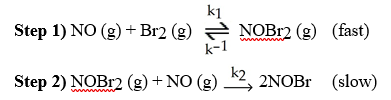A possible mechanism for the overall reaction

Br2 (g) + 2NO (g) → 2NOBr (g) is [see image]

What is the rate determining step for this reaction?
A) step 2
B) reverse of step 1
C) both steps 1 and 2
D) step 1
E) reverse of step 2

A

34

Which of the following is true?
A) If we know that a reaction is an elementary reaction, then we know its rate law.
B) The rate-determining step of a reaction is the rate of the fastest elementary step of its mechanism.
C) Since intermediate compounds can be formed, the chemical equations for the elementary reactions in a multistep mechanism do not always have to add to give the chemical equation of the overall process.
D) In a reaction mechanism, an intermediate is identical to an activated complex.
E) All of the above statements are true.

A

35

Of the following, ________ will lower the activation energy for a reaction.
A) increasing the concentrations of reactants
B) raising the temperature of the reaction
C) adding a catalyst for the reaction
D) removing products as the reaction proceeds
E) increasing the pressure

C

36

The rate law of the overall reaction

A + B → C

is rate = k[A]2. Which of the following will not increase the rate of the reaction?
A) increasing the concentration of reactant A
B) increasing the concentration of reactant B
C) increasing the temperature of the reaction
D) adding a catalyst for the reaction
E) All of these will increase the rate.

B

37

A catalyst can ________ the rate of a reaction by providing an alternative pathway with a ________ activation energy
A) decrease, higher
B) increase, lower
C) decrease, lower
D) increase, higher
E) decrease, constant

B

38

The primary source of the specificity of enzymes is ________.
A) their polarity, which matches that of their specific substrate
B) their delocalized electron cloud
C) their bonded transition metal, which is specific to the target substrate
D) their locations within the cell
E) their shape, which relates to the lock-and-key model

E

39

________ are used in automotive catalytic converters.
A) Heterogeneous catalysts
B) Homogeneous catalysts
C) Enzymes
D) Noble gases
E) Nonmetal oxides

A

40

The enzyme ________ converts nitrogen into ammonia.
A) oxygenase
B) hydrogenase
C) oxidase
D) nitroglycerine
E) nitrogenase

E

41

Fe and Mo are transition metals found in the cofactor active site of ________.
A) hydrogenase
B) nitrogenase
C) oxidase
D) nitroglycerine
E) oxygenase

B

42

Which of the following statements describes why nitrogen fixation is a difficult process?
A) there is so little nitrogen in the atmosphere
B) nitrogen is very unreactive, largely due to its triple bond
C) nitrogen exists in the atmosphere primarily as its oxides which are very unreactive
D) of the high polarity of nitrogen molecules preventing them from dissolving in biological fluids, such as those inside cells
E) of the extreme toxicity of nitrogen

B

43

Consider the following reaction:

3A → 2B

The average rate of appearance of B is given by Δ[B]/Δt. Comparing the rate of appearance of B and the rate of disappearance of A, we get Δ[B]/Δt = ________ x (-Δ[A]/Δt).
A) -2/3
B) +2/3
C) -3/2
D) +1
E) +3/2

B

44

Which substance in the reaction below either appears or disappears the fastest?

4NH3 + 7O2 → 4NO2 + 6H2O

A) NH3
B) O2
C) NO2
D) H2O
E) The rates of appearance/disappearance are the same for all of these.

B

45

Consider the following reaction:

A → B

The average rate of appearance of B is given by Δ[B]Δt. Comparing the rate of appearance of B and the rate of disappearance of A, we get Δ[B]/Δt = ________ x (-Δ[A]/Δt).
A) +1
B) +2
C) -1
D) +1/2
E) -1/2

A

46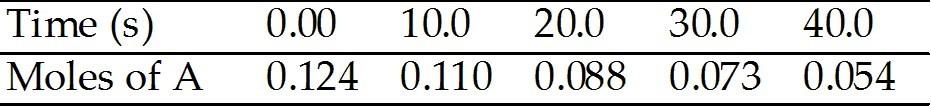A flask is charged with 0.124 mol of A and allowed to react to form B according to the reaction A(g) →B(g). The following data are obtained for [A] as the reaction proceeds:

The average rate of disappearance of A between 10 s and 20 s is ________ mol/s.
A) 2.2 × 10-3
B) 1.1 × 10-3
C) 4.4 × 10-3
D) 454
E) 9.90 × 10-3

A

47A flask is charged with 0.124 mol of A and allowed to react to form B according to the reaction A(g) →B(g). The following data are obtained for [A] as the reaction proceeds:

The average rate of disappearance of A between 20 s and 40 s is ________ mol/s.
A) 8.5 × 10-4
B) 1.7 × 10-3
C) 590
D) 7.1 × 10-3
E) 1.4 × 10-3

B

48A flask is charged with 0.124 mol of A and allowed to react to form B according to the reaction A(g) →B(g). The following data are obtained for [A] as the reaction proceeds:

The average rate of appearance of B between 20 s and 30 s is ________ mol/s.
A) +1.5 × 10-3
B) +5.0 × 10-4
C) -1.5 × 10-3
D) +7.3 × 10-3
E) -7.3 × 10-3

A

49A flask is charged with 0.124 mol of A and allowed to react to form B according to the reaction A(g) →B(g). The following data are obtained for [A] as the reaction proceeds:

The average rate disappearance of A between 20 s and 30 s is ________ mol/s.
A) 5.0 × 10-4
B) 1.6 × 10-2
C) 1.5 × 10-3
D) 670
E) 0.15

C

50A flask is charged with 0.124 mol of A and allowed to react to form B according to the reaction A(g) →B(g). The following data are obtained for [A] as the reaction proceeds:

How many moles of B are present at 40. s?
A) 0.18
B) 0.070
C) 0.124
D) 0.054
E) 7.8 × 10-3

B

51A flask is charged with 0.124 mol of A and allowed to react to form B according to the reaction A(g) →B(g). The following data are obtained for [A] as the reaction proceeds:

How many moles of B are present at 30 s?
A) 2.4 × 10-3
B) 0.15
C) 0.073
D) 1.7 × 10-3
E) 0.051

E

52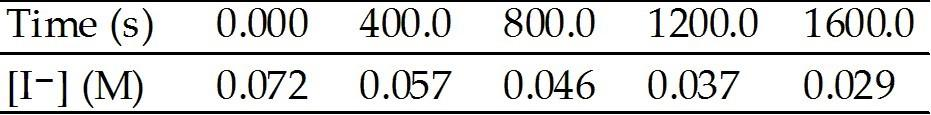The peroxydisulfate ion (S2O82-) reacts with the iodide ion in aqueous solution via the reaction:

S2O82- (aq) + 3I- → 2SO42- (aq) + I3- (aq)

An aqueous solution containing 0.050 M of S2O82- ion and 0.072 M of I- is prepared, and the progress of the reaction followed by measuring [I-]. The data obtained is given in the table below.

The average rate of disappearance of I- between 400.0 s and 800.0 s is ________ M/s.
A) 2.8 × 10-5
B) 1.4 × 10-5
C) 5.8 × 10-5
D) 3.6 × 104
E) 2.6 × 10-4

A

53The peroxydisulfate ion (S2O82-) reacts with the iodide ion in aqueous solution via the reaction:

S2O82- (aq) + 3I- → 2SO42- (aq) + I3- (aq)

An aqueous solution containing 0.050 M of S2O82- ion and 0.072 M of I- is prepared, and the progress of the reaction followed by measuring [I-]. The data obtained is given in the table below.

What is the average rate of disappearance (M/s) of I- in the initial 800.0 s?
A) 2.8
B) 2.8 × 10-5
C) 3.3 × 10-5
D) 2.6 × 10-2
E) 3.1 × 104

C

54The peroxydisulfate ion (S2O82-) reacts with the iodide ion in aqueous solution via the reaction:

S2O82- (aq) + 3I- → 2SO42- (aq) + I3- (aq)

An aqueous solution containing 0.050 M of S2O82- ion and 0.072 M of I- is prepared, and the progress of the reaction followed by measuring [I-]. The data obtained is given in the table below.

What is the average rate of disappearance (M/s) of I- between 800.0 s and 1200.0 s?
A) 2.8 × 10-5
B) 3.3 × 10-5
C) 5.8 × 104
D) 2.3 × 10-5
E) 2.6 × 10-4

D

55The peroxydisulfate ion (S2O82-) reacts with the iodide ion in aqueous solution via the reaction:

S2O82- (aq) + 3I- → 2SO42- (aq) + I3- (aq)

An aqueous solution containing 0.050 M of S2O82- ion and 0.072 M of I- is prepared, and the progress of the reaction followed by measuring [I-]. The data obtained is given in the table below.

What is the concentration (M) of S2O82- remaining at 1200 s?
A) +0.035
B) +0.038
C) -0.038
D) +0.012
E) -0.012

B

56The peroxydisulfate ion (S2O82-) reacts with the iodide ion in aqueous solution via the reaction:

S2O82- (aq) + 3I- → 2SO42- (aq) + I3- (aq)

An aqueous solution containing 0.050 M of S2O82- ion and 0.072 M of I- is prepared, and the progress of the reaction followed by measuring [I-]. The data obtained is given in the table below.

The concentration of S2O82- remaining at 800 s is ________ M.
A) 0.046
B) 0.076
C) 4.00 × 10-3
D) 0.015
E) 0.041

E

57The peroxydisulfate ion (S2O82-) reacts with the iodide ion in aqueous solution via the reaction:

S2O82- (aq) + 3I- → 2SO42- (aq) + I3- (aq)

An aqueous solution containing 0.050 M of S2O82- ion and 0.072 M of I- is prepared, and the progress of the reaction followed by measuring [I-]. The data obtained is given in the table below.

What is the concentration (M) of S2O82- remaining at T=0?
A) 0.072
B) 0.022
C) 0.15
D) 0.050
E) no S2O82- is remaining at T=0

D

58

At elevated temperatures, dinitrogen pentoxide decomposes to nitrogen dioxide and oxygen:

2N2O5(g) → 4NO2 (g) + O2 (g)

When the rate of formation of NO2 is 5.5 × 10-4 M/s, the rate of decomposition of N2O5 is ________ M/s.
A) 2.2 × 10-3
B) 1.4 × 10-4
C) 10.1 × 10-4
D) 2.8 × 10-4
E) 5.5 × 10-4

D

59

At elevated temperatures, methylisonitrile (CH3NC) isomerizes to acetonitrile (CH3CN):

CH3NC (g) → CH3CN (g)

At the start of the experiment, there are 0.200 mol of reactant (CH3NC) and 0 mol of product (CH3CN) in the reaction vessel. After 25 min of reaction, 0.108 mol of reactant (CH3NC) remain. The average rate of decomposition of methyl isonitrile, CH3NC, in this 25 min period is ________ mol/min.
A) 3.7 × 10-3
B) 0.092
C) 2.3
D) 4.3 × 10-3
E) 0.54

A

60

A reaction was found to be second order in carbon monoxide concentration. The rate of the reaction ________ if the [CO] is doubled, with everything else kept the same.
A) doubles
B) remains unchanged
C) triples
D) increases by a factor of 4
E) is reduced by a factor of 2

D

61

) If the rate law for the reaction

2A + 3B → products

is first order in A and second order in B, then the rate law is
A) k[A][B]
B) k[A]2[B]3
C) k[A][B]2
D) k[A]2[B]
E) k[A]2[B]2

C

62

If the rate law for the reaction

A + 3B → C + 2D

is zero order in A and first order in B, then the rate law is
A) k[A]
B) k[A][B]3
C) k[B]
D) k[A][B]
E) k[A]/[B]3

C

63

The overall order of a reaction is 1. The units of the rate constant for the reaction are ________.
A) M/s
B) M-1s-1
C) 1/s
D) 1/M
E) s/M2

C

64

The overall order of a reaction is 2. The units of the rate constant for the reaction are ________.
A) M/s
B) M-1s-1
C) 1/s
D) 1/M
E) s/M2

B

65

It was determined experimentally that the reaction rate doubled when the concentration of B was doubled in the following reaction:

A + B → C

The reaction is ________ order in B.
A) zero
B) first
C) second
D) third
E) one-half

B

66

It was determined experimentally that the reaction rate remained the same when the concentration of A was doubled in the following reaction:

A + B → C

The reaction is ________ order in A.
A) zero
B) first
C) second
D) third
E) one-half

A

67

A reaction was found to be third order in A. Increasing the concentration of A by a factor of 3 will cause the reaction rate to ________.
A) remain constant
B) increase by a factor of 27
C) increase by a factor of 9
D) triple
E) decrease by a factor of the cube root of 3

B

68

A reaction was found to be zero order in A. Increasing the concentration of A by a factor of 3 will cause the reaction rate to ________.
A) remain constant
B) increase by a factor of 27
C) increase by a factor of 9
D) triple
E) decrease by a factor of the cube root of 3

A

69The data in the table below were obtained for the reaction:

A + B → C

What is the order of the reaction in A?
A) 1
B) 2
C) 3
D) 4
E) 0

B

70The data in the table below were obtained for the reaction:

A + B → C

What is the order of the reaction in B?
A) 1
B) 2
C) 3
D) 4
E) 0

E

71The data in the table below were obtained for the reaction:

A + B → C

What is the overall order of the reaction?
A) 1
B) 2
C) 3
D) 4
E) 0

B

72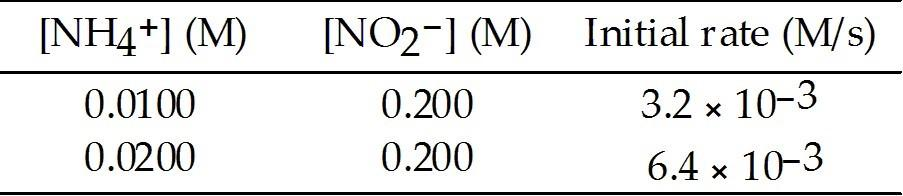The following reaction occurs in aqueous solution:

NH4+ (aq) + NO2- (aq) → N2 (g) + 2H2O (l)

The data below is obtained at 25 °C.

The order of the reaction in NH4+ is ________.
A) -2
B) -1
C) +2
D) +1
E) 0

D

73

For a zero-order reaction, a plot of ________ versus ________ is linear.
A) ln [A]t, 1/t
B) [A]t, t
C) ln [A]t, t
D) 1/[A]t, t
E) t, 1/[A]t

B

74

The half-life of a first-order reaction is 13 min. If the initial concentration of reactant is 0.085 M, it takes ________ min for it to decrease to 0.055 M.
A) 8.2
B) 11
C) 3.6
D) 0.048
E) 8.4

A

75

The half-life of a first-order reaction is 13 min. If the initial concentration of reactant is 0.13 M, it takes ________ min for it to decrease to 0.085 M.
A) 12
B) 10
C) 8.0
D) 11
E) 7.0

C

76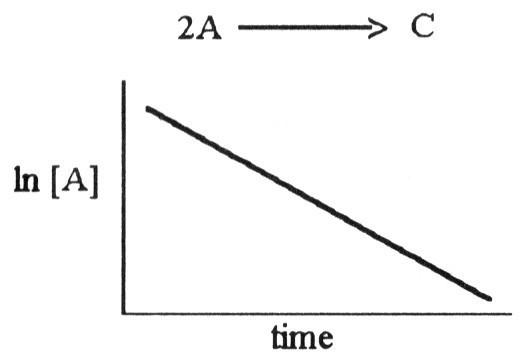The graph shown below depicts the relationship between concentration and time for the following chemical reaction.

The slope of this line is equal to ________.
A) k
B) -1/k
C) ln [A]o
D) -k
E) 1/k

D

77

The reaction below is first order in [H2O2]:

2H2O2 (l) → 2H2O (l) + O2 (g)

A solution originally at 0.600 M H2O2 is found to be 0.075 M after 54 min. The half-life for this reaction is ________ min.
A) 6.8
B) 18
C) 14
D) 28
E) 54

B

78

A second-order reaction has a half-life of 18 s when the initial concentration of reactant is 0.71 M. The rate constant for this reaction is ________ M-1s-1.
A) 7.8 × 10-2
B) 3.8 × 10-2
C) 2.0 × 10-2
D) 1.3
E) 18

A

79

A second-order reaction has a half-life of 12 s when the initial concentration of reactant is 0.98 M. The rate constant for this reaction is ________ M-1s-1.
A) 12
B) 2.0 × 10-2
C) 8.5 × 10-2
D) 4.3 × 10-2
E) 4.3

C

80

Of the following, only ________ is a valid unit for reaction rate.
A) M/s
B) mol/g
C) mmol/mL
D) g/L
E) atm/g

A

81

The overall reaction below is ________ and the elementary reaction is considered ________.

AB → A + B

A) unimolecular, rare
B) bimolecular, common
C) unimolecular, common
D) termolecular, rare
E) bimolecular, rare

C

82

Of the units below, ________ are appropriate for a third-order reaction rate constant.
A) M-2s-1
B) M s-1
C) s-1
D) M-1s-1
E) mol/L

A

83

The rate law for a reaction is

rate = k[A][B]

Which one of the following statements is false?
A) The reaction is first order overall.
B) The reaction is first order in [A].
C) The reaction is first order in [B].
D) k is the reaction rate constant
E) If [A] is doubled, the reaction rate will increase by a factor of 2.

A

84

Nitrogen dioxide decomposes to nitric oxide and oxygen via the reaction:

2NO2 → 2NO + O2

In a particular experiment at 300 °C, [N ] drops from 0.0143 to 0.00701 M in 261 s. The rate of disappearance of NO2 for this period is ________ M/s.
A) 2.79 × 10-5
B) -8.16 × 10-5
C) 5.59 × 10-5
D) 1.40 × 10-5
E) 35,800

A

85

At elevated temperatures, dinitrogen pentoxide decomposes to nitrogen dioxide and oxygen:

2N2O5(g) → 4NO2 (g) + O2 (g)

When the rate of formation of O2 is 3.6 × 10-3 M/s, the rate of decomposition of N2O5 is
A) 4.4 × 10-4
B) 3.6 × 10-3
C) 1.8 × 10-3
D) 1.4 × 10-2
E) 0.00090

A

86

The rate of disappearance of HBr in the gas phase reaction

2HBr (g) → H2 (g) + Br2 (g)

is 0.190 M s-1 at 150 °C. The rate of appearance of Br2 is ________ M s-1.
A) 2.63
B) 0.095
C) 0.0361
D) 0.380
E) 0.436

B

87

The rate of disappearance of HBr in the gas phase reaction

2HBr (g) → H2 (g) + Br2 (g)

is 0.140 M s-1 at 150 °C. The rate of reaction is ________ M s-1.
A) 3.57
B) 0.0700
C) 0.0196
D) 0.280
E) 0.0860

B

88

During the combustion of ethylene gas, C2H4, carbon dioxide is produced and the rate of disappearance of O2 is 0.13 M s-1. What is the rate of appearance (M s-1) of CO2?
A) 0.043
B) 0.39
C) 0.087
D) 0.20
E) 0.26

C

89

The combustion of ethylene proceeds by the reaction

C2H4 (g) + 3O2 (g) → 2CO2 (g) + 2H2O (g)

When the rate of disappearance of O2 is 0.13 M s-1, the rate of disappearance of C2H4 is ________ M s-1
A) 0.087
B) 0.043
C) 0.39
D) 0.20
E) 0.26

B

90

Nitrogen dioxide decomposes to nitric oxide and oxygen via the reaction:

2NO2 (g) → 2NO (g) + O2 (g)

In a particular experiment at 300 °C, [NO2] drops from 0.0100 to 0.00800 M in 100 s. The rate of appearance of O2 for this period is ________ M/s.
A) 1.0 × 10-5
B) 2.0 × 10-5
C) 4.0 × 10-5
D) 2.0 × 10-3
E) 4.0 × 10-3

A

91

At elevated temperatures, methylisonitrile (CH3NC) isomerizes to acetonitrile (CH3CN):

CH3NC (g) → CH3CN (g)

At the start of an experiment, there are 0.200 mol of reactant and 0 mol of product in the reaction vessel. After 25 min, 0.121 mol of reactant (CH3NC) remain. There are ________ mol of product (CH3CN) in the reaction vessel.
A) 0.022
B) 0.121
C) 0.200
D) 0.321
E) 0.079

E

92

A compound decomposes by a first-order process. If 13% of the compound decomposes in 60 minutes, the half-life of the compound is ________ min.
A) 299
B) 20
C) 12
D) -18
E) -5

A

93

What value is represented by the y-intercept of the line drawn from plotting [A] vs. time in a linear graphical representation of a zero-order reaction?
A) ln[A]0
B) 1/[A]0
C) [A]0
D) [A]0 2
E) [A]/k

C

94

The rate constant for a particular zero-order reaction is 0.075 M s-1. If the initial concentration of reactant is 0.537 M it takes ________ s for the concentration to decrease to 0.100 M.
A) 5.8
B) -5.8
C) -0.047
D) 7.2
E) 0.040

A

95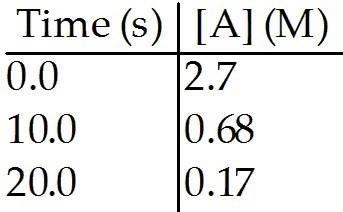The reaction A → B is first order in [A]. Consider the following data.

The rate constant for this reaction is ________ s-1.
A) 0.14
B) 0.030
C) 0.013
D) 3.0
E) 3.1 × 10-3

A

96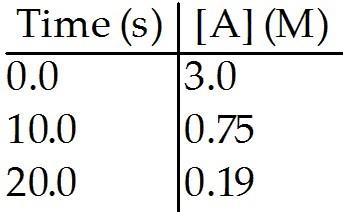The reaction A → B is first order in [A]. Consider the following data.

What is the half-life, in seconds, of this reaction?
A) 5.0
B) 0.97
C) 7.1
D) 3.0
E) 0.14

A

97

What is the rate constant ( s-1) of a first-order process that has a half-life of 550 s?
A) 3.81 × 102
B) 7.94 × 102
C) -9.09 × 10-4
D) 1.26 × 10-3
E) 1.82 × 10-3

D

98

The following reaction is second order in [A] and the rate constant is 0.039 M-1s-1:

A → B

What is the initial concentration (M) of A if at 28 s the concentration of A was 0.26 M?
A) 2.8
B) -1.2
C) 0.36
D) -1.5
E) -0.83

C

99

What is the half-life of an unknown compound if 17.0% of the compound decomposes in 150 minutes via a first-order process?
A) 59 minutes
B) 40 minutes
C) 1116 minutes
D) 279 minutes
E) 558 minutes

E

100

The isomerization of methylisonitrile to acetonitrile

CH3NC (g) → CH3CN (g)

is first order in CH3NC. The rate constant for the reaction is 9.45 × 10-5 s-1 at 478 K. The half-life of the reaction when the initial [CH3NC] is 0.030 M is ________ s.
A) 1.06 × 104
B) 5.29 × 103
C) 3.53 × 105
D) 7.33 × 103
E) 1.36 × 10-4

D

101

The elementary reaction

2NO2 (g) → 2NO (g) + O2 (g)

is second order in NO2 and the rate constant at 660 K is 5.23 M-1s-1. The reaction half-life at this temperature when [NO2]0 = 0.45 M is ________ s.
A) 2.4
B) 7.6
C) 0.19
D) 0.13
E) 0.42

E

102

The isomerization of methylisonitrile to acetonitrile

CH3NC (g) → CH3CN (g)

is first order in CH3NC. The half-life of the reaction is 2.70 × 104 s at 463 K. The rate constant is ________ s-1 when the initial [CH3NC] is 0.030 M.
A) 3.90 × 104
B) 1.23 × 10-3
C) 2.57 × 10-5
D) 8.10 × 102
E) 1.25 × 107

C

103

The decomposition of in solution in carbon tetrachloride proceeds via the reaction

2N2O5 (soln) → 4NO2 (soln) + O2 (soln)

The reaction is first order and has a rate constant of 4.82 × 10-3 s-1 at 64 °C. If the reaction is initiated with 0.072 mol in a 1.00-L vessel, how many moles remain after 151 s?
A) 0.067
B) 0.074
C) 0.035
D) 9.6
E) 1.6 × 103

C

104

SO2Cl2 decomposes in the gas phase by the reaction

SO2Cl2 (g) → SO2 (g) + Cl2 (g)

The reaction is first order in SO2Cl2 and the rate constant is 3.0 × 10-6 s-1 at 600 K. A vessel is charged with 3.6 atm of SO2Cl2 at 600 K. The partial pressure of SO2Cl2 at 3.0 x 105 s is ________ atm.
A) 0.85
B) 3.2
C) 1.5
D) 0.19
E) 9.3 × 104

C

105

The rate constant for a particular second-order reaction is 0.47 M-1s-1. If the initial concentration of reactant is 0.25 mol/L, it takes ________ s for the concentration to decrease to 0.20 mol/L.
A) 2.1
B) 1.4
C) 1.0
D) 0.47
E) 0.20

A

106

The reaction

2NOBr (g) → 2 NO (g) + Br2 (g)

is a second-order reaction with a rate constant of 0.80 M-1s-1 at 11 °C. If the initial concentration of NOBr is 0.0440 M, the concentration of NOBr after 6.0 seconds is ________.
A) 0.0276 M
B) 0.0324 M
C) 0.0363 M
D) 0.0348 M
E) 0.0402 M

C

107

A first-order reaction has a rate constant of 0.33 min-1. It takes ________ min for the reactant concentration to decrease from 0.13 M to 0.066 M.
A) 0.085
B) 0.13
C) 0.89
D) 2.4
E) 2.1

E

108

The initial concentration of reactant in a first-order reaction is 0.27 M and has a rate constant of 0.75 s-1. What is the concentration (mol/L) of reactant after 0.75 s?
A) 0.99
B) 1.7
C) 0.074
D) 0.15
E) 0.47

D

109

The rate constant for a second-order reaction is 0.13 M-1s-1. If the initial concentration of reactant is 0.26 mol/L, it takes ________ s for the concentration to decrease to 0.07 mol/L.
A) 0.017
B) 1.4
C) 14
D) 80.
E) 10.

D

110

At elevated temperatures, nitrogen dioxide decomposes to nitrogen oxide and oxygen:

NO2 (g) → NO (g) + 1/2 O2 (g)
The reaction is second order in NO2 with a rate constant of 0.543 M-1s-1 at 300 °C. If the initial [NO2] is 0.260 M, it will take ________ s for the concentration to drop to 0.075 M.
A) 3.34
B) 0.0880
C) 2.29
D) 0.299
E) 17.5

E

111

A particular first-order reaction has a rate constant of 1.35 × 102 s-1 at 25.0 °C. What is the magnitude of k at 650 °C if Ea = 55.5 kJ/mol?
A) 1.92 × 103
B) 1.95 × 104
C) 358
D) 3.48 × 1073
E) 1.35 ×

A

112

What is the magnitude of k for a first-order reaction at 75.0 °C if Ea = 95.1 kJ/mol and rate constant is 1.35 × 102 s-1 at 25.0 °C?
A) 3.36 × 104
B) 3.85 ×
C) 745
D) 4.28 ×
E) 1.36 ×

A

113

Define Beer's Law.

the direct relationship of absorbed light to the concentration of the substance absorbing the light

114

Write the rate law for the reaction:

aA → bB + cC.

rate = k[A]m

115

If a rate law is first order (reactant), doubling the reactant ________ the reaction rate.

doubles

116

The Earth's ozone layer is located in the ________.

stratosphere

117

According to the collision model, reaction rates are affected by reactant ________ and ________.

concentration, temperature

118

Define activation energy.

the minimum energy required to initiate a chemical reaction

119

The rate of a chemical reaction should ________ as the temperature rises.

increase

120

The rate-determining step is the ________ elementary step.

slowest

121

A unimolecular elementary reaction involves ________ reactant molecule(s).

one

122

Define a homogeneous catalyst.

a catalyst that is present in the same phase as the reacting molecules

123

Define a heterogeneous catalyst.

a catalyst present in a different phase from the reacting molecules

124

Biological catalysts are referred to as ________.

enzymes

125

A ________ is a substance that speeds up a chemical reaction without itself being consumed in the reaction.

catalyst

126

Rates of reaction can be positive or negative.

false

127

The instantaneous rate of a reaction can be read directly from the graph of molarity versus time at any point on the graph.

false

128

The overall reaction order is the sum of the orders of each reactant in the rate law.

true

129

Units of the rate constant of a reaction are independent of the overall reaction order.

false

130

The concentration of reactants or products at any time during the reaction can be calculated from the integrated rate law.

true

131

The rate of a second order reaction can depend on the concentrations of more than one reactant.

true

132

The half-life for a first order rate law depends on the starting concentration.

false

133

The rate limiting step in a reaction is the slowest step in the reaction sequence.

true

134

Heterogeneous catalysts have different phases from reactants.

true